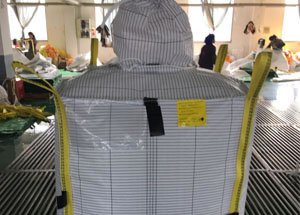# The calculation method of the weight of the bulk bagsThe quality of the woven bag is inseparable from the weight of the gram. Generally speaking, the higher the weight, the thicker the bag, the better the quality. The measure is in weight. The base bag of the bulk bags is calculated by the square meter, which is generally 160 grams per square, 180 grams, 190 grams, 200 grams per square. If you cut the piece down, measure the size, and then weigh the weight, you can calculate the square of the square of the square. The weight of the woven bag directly determines the thickness of the woven bag. The calculation formula is as follows: single bag weight = length * width * 2 * square gram weight.
For example, thickness 60 microns, size 180 * 270cm
weight is volume * density, volume 0.006 * 180 * 2 * 270 = 583.2 density
PE is 0.917
583.2 * 0.917 = 531.2952 grams
There are relatively cumbersome calculation method: (number of latitudes) /2000.911=square gram weight